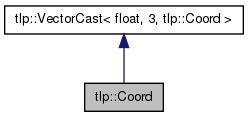Overview Modules Class Hierarchy Classes Members
tlp::Coord Class Reference

This class represents a point in 3-D space, with float precision. More...

`#include <Coord.h>`

Inheritance diagram for tlp::Coord:[legend]
Collaboration diagram for tlp::Coord:[legend]

List of all members.

## Public Member Functions

• Coord (const tlp::Vector< float, 3 > &v)
Copy constructor.
• Coord (const float xx=0, const float yy=0, const float zz=0)
Default constructor. The values default on 0 if not filled.
• void set (const float xx=0.f, const float yy=0.f, const float zz=0.f)
Sets the value of the X, Y, and Z value of this Coordinate.
• void set (const Coord &c)
Copy the values of the parameter coordinate into this coordinate.
• void setX (float xx)
• void setY (float yy)
• void setZ (float zz)
• float getX () const
• float getY () const
• float getZ () const
• void get (float &xx, float &yy, float &zz) const
Modifies the values of the parameters to the vaules of this Coordinate's composants.

## Detailed Description

This class represents a point in 3-D space, with float precision.

## Constructor & Destructor Documentation

 tlp::Coord::Coord ( const tlp::Vector< float, 3 > & v ) ` [inline, explicit]`

Copy constructor.

Parameters:
 v A tlp::Coord to copy.
 tlp::Coord::Coord ( const float xx = `0`, const float yy = `0`, const float zz = `0` ) ` [inline, explicit]`

Default constructor. The values default on 0 if not filled.

Parameters:
 xx The X value of this Coord. Defaults to 0. yy The Y value of this Coord. Defaults to 0. zz The Z value of this Coord. Defaults to 0.

## Member Function Documentation

 void tlp::Coord::get ( float & xx, float & yy, float & zz ) const` [inline]`

Modifies the values of the parameters to the vaules of this Coordinate's composants.

Parameters:
 xx This Coord's X value. yy This Coord's Y value. zz This Coord's Z value.
Returns:
void
 float tlp::Coord::getX ( ) const` [inline]`
 float tlp::Coord::getY ( ) const` [inline]`
 float tlp::Coord::getZ ( ) const` [inline]`
 void tlp::Coord::set ( const Coord & c ) ` [inline]`

Copy the values of the parameter coordinate into this coordinate.

Parameters:
 c A Coord to copy.
Returns:
void
 void tlp::Coord::set ( const float xx = `0.f`, const float yy = `0.f`, const float zz = `0.f` ) ` [inline]`

Sets the value of the X, Y, and Z value of this Coordinate.

Parameters:
 xx The X value of this Coord. Defaults to 0.f. yy The Y value of this Coord. Defaults to 0.f. zz The Z value of this Coord. Defaults to 0.f.
Returns:
void
 void tlp::Coord::setX ( float xx ) ` [inline]`
 void tlp::Coord::setY ( float yy ) ` [inline]`
 void tlp::Coord::setZ ( float zz ) ` [inline]`

 Tulip Software by LaBRI Visualization Team    2001 - 2012